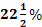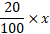304 North Cardinal St.
Dorchester Center, MA 02124

# Important Profit and Loss Question for Bank/IBPS/SBI PO Clerk/SSC/State Private Jobs

-

Profit and loss question are very important questions if you are preparing for banking IBPS, SBI PO clerk and other important quantitative exam then profit and loss questions are very important. As you can expect 3 to 4 questions in quantitative section. If you practice a lot then it is easy to score in these questions.

P&L statement, also referred as an income statement, measures business revenue (income or sales) and expenses during a given time period.

Put another way, a profit and loss statement tells you whether or not your business is making money.  Small business owners can use a P&L statement to assess business performance, identifying room for improvement and new strategies for growth.

Generally P&L statement is assessed over the following common time periods:

• Monthly
• Quarterly
• Annually

## Important Profit & Loss Topics for Bank Exams

The table below highlights the Profit & Loss syllabus for bank exams:

### Profit & Loss Tricks for Bank Exams

Now, we will learn some tips/tricks to solve any Banking Question related to Profit & Loss.

• Cost Price = ⦃ 100/(100+Profit %)⦄ x Selling Price
• Cost Price = ⦃ 100/(100-Loss %)⦄ x Selling Price
• Selling Price = ⦃(100+Profit%)/100⦄ x Cost Price
• Selling Price = ⦃(100-Loss%)/100⦄ x Cost Price

Tips and Tricks to solve Profit Loss Questions :

-

Tip 1: If the selling price of two items is same but one item is sold at x% profit and another is sold at x% loss, then loss %

(In this case, there is always a loss.)

Example

If Ram sold two T.V. in the same price. He sold one T.V. at 10% profit and another at 10% loss. Find the % loss/ profit on total. Solution:

Loss % = 1%

Tip 2: If a shopkeeper sells his goods at cost price but uses a weight of ‘x’ gram instead of ‘y’ gram to gain ‘a%’ profit

Example A dishonest dealer profess to sell his goods at C.P. and uses a weight of 875 gram instead of 1 kg. to gain X% profit. Find the value of X. Solution:

Tip 3:

If a shopkeeper sells his goods at ‘x%’ loss on cost price but uses ‘y’ gm instead of ‘z’ gm, then, his % profit or loss

Example A dishonest dealer sells goods at 25/4% loss on cost price but uses the weight of 14 gram instead of 16 gram. What is his % profit or loss? Solution:

=7.14%

Tip 4:

If the selling price of ‘X’ pens is equal to the cost price of Y’ pens. Then

Note: If the Sign is +ve, there is gain. If the sign is -ve, there is a loss.

Example
If the selling price of 60 eggs is equal to the cost price of 75 eggs. What is the profit % of that eggs?

Profit % = 25%

Practice Questions

Q.1. A man buys an article for Rs. 27.50 and sells it for Rs. 28.60. Find his gain percent.

Solution:

C.P = Rs. 27.50, S.P. = Rs. 28.60

So, Gain = Rs. (28.60 – 27.50) = Rs. 1.10.

Q.2. If a radio is purchased for Rs. 490 and sold for Rs. 466.50, Find the loss percent.

Solution:

C.P. = Rs. 490, S.P = Rs. 465.50

Loss = Rs. (490 – 465.50) = Rs. 24.50

Q.3. Find S.P, when

(i) C.P = Rs. 56.25, Gain = 20%

(ii) C.P. = Rs. 80.40, Loss = 5%

Solution:. (i) S.P = 120% of Rs. 56.25

Solution: (ii) S.P = 85% of Rs. 80.40

Q.4. Find C.P., when

(i) S.P. = Rs. 40.60, Gain = 16%

(ii) S.P = Rs. 51.70, Loss = 12%

Solution:(i) C.P

Solution: (ii) C.P

Q.5. A person incurs 5% loss by selling a watch for Rs. 1140. At what price should the watch be sold to earn 5% profit ?

Solution:

Let the new S.P be Rs. X. Then,

(100 – loss%) : (1st S.P.) = (100 + gain%) : ( 2nd SP.)

Q.6. If the cost price is 96% of the selling price, then what is the profit percent?

Solution:

Let S.P = Rs. 100. Then, CP = Rs. 96; Profit = Rs.4.

Q.7. By selling an article for Rs. 100, a man gains Rs. 15. Then, his gain% is :

Solution

S.P = Rs. 100, gain = Rs. 15.

∴ C.P = Rs. (100-15)= Rs. 85.

Q.8. When a commodity is sold for Rs.34.80, there is a loss of 25%. What is cost price of the commodity?

Solution

Q.9. A shopkeeper expects a gain ofon his cost price. If in a weak, his sale was of Rs. 392, what was his profit?

Solution

Profit = Rs. (392 – 320) = Rs. 72.

Q.10. The sale price of an article including the sales tax is Rs. 616. The rate of sales tax is 10%. If the shopkeeper has made a profit of 12%, then the cost price of the article is:

Solution

110% of S.P = 616

Q.11. Saransh purchased 120 reams of paper at Ra. 80 per ream. He spent Rs. 280 on transportation, paid octroi at the rate of 40 paise per ream and paid Rs. 72 to the colie. If he wants to have a gain 8%, what must be the selling price per ream ?

Solution

Total investment

S.P of 120 reams = 108% of Rs. 10000 = Rs. 10800.

∴ S.P per ream

Q.12. A person bought 20 litres of milk at the rate of Rs. 8 per litre. He got it churned after spending Rs. 10 and 5 kg of cream and 20 litres of toned milk were obtained. If he sold the cream at Rs. 30 per kg and toned milk at Rs 4 per litre, his profit in the transaction is:

Solution

Investment = Rs. (20×8+10)=Rs. 170. Receipt = Rs. (30×5+20×4)=Rs.230.

Q.13. Jacob bought a scooter for a certain sum of money. He spent 10% of the cost on repairs and sold the scooter for a profit of Rs. 1100. How much did he spend on repairs if he made a profit of 20%?

Solution

Let the C.P. be Rs. x. Then, 20% of x = 1100 →=1100→ x = 5500.

C.P. = Rs. 5500, Expenditure on repairs = 10%

∴ Expenditure on repairs = Rs. (5500 – 5000)=Rs.500.

Q.14. A manufacture undertakes to supply 2000 pieces of a particular component at Rs. 25 per piece. According to his estimates, even if 5% fail to pass the quality tests, then he will make a profit of 25%. However, as it turned out. 50% of the components were rejected. What is the loss to the manufacturer?

Solution

Total cost incurred

Loss to the manufacturer = Rs. [38000 – (25×1000)]=Rs.13000.

Q.15. A trader buys a chair for Rs. 600 and sells it for Rs. 765 at a credit of 4 months. Reckoning money worth 6% p.a., his gain percent is

Solution

Solution

if you face any problem while solving profit and loss questions, you can ask me in the comment section.

Q :  The cost price of 60 articles is same as the selling price of x articles. If there is a proﬁt of 20%, then what is the value of x?

(A) 15

(B) 50

(C) 30

(D) 80

Q :  While selling an article for Rs 18450, a person suffered a loss of 50%. At what price he should have sold the article (in Rs) to earn a profit of 50%?

(A) 13837

(B) 52000

(C) 56775

(D) 55350

Q :  If a vendor sells apples at Rs 170 per kg he suffers 15 % loss. If he sells at Rs 230 per kg, then what is his profit percentage?

(A) 5

(B) 20

(C) 15

(D) 10

Q :  A trader sells two items at the rate of Rs 400 each. If he gains 15% on one and losses 15% on other, then what is the value (in Rs) of loss?

(A) 24.36

(B) 22.14

(C) 20.25

Q :  Vinayak bought an item and sold it at a loss of 5%. If he had bought it for 10% less and sold it for Rs.390 more, he would have had a profit of 20%, find the cost price of item.

(A) Rs. 3300

(B) Rs. 3600

(C) Rs. 3200

(D) Rs. 3000

D : 3000

Q :  A car worth Rs 30,000 was sold by A to B at 10 % profit. B sold the car back to A at 5 % loss. In the entire transaction.

(A) A gain Rs 1500

(B) A loss Rs 1500

(C) A gain Rs. 1650

(D) A gain Rs. 1850

Q :  Vivek buys 3 shirts at an average price of Rs. 1250. If he buys 2 more shirts at an average price of Rs. 1450. What will be the average price (in Rs.) of all the 5 shirts he buys?

(A) 1350

(B) 1390

(C) 1330

(D) 1310

-